# Scientific Notation Scientific Notation In science we deal

• Slides: 17Scientific NotationScientific Notation In science, we deal with some very LARGE numbers: 1 mole = 60200000000000 In science, we deal with some very SMALL numbers: Mass of an electron = 0. 000000000000000091 kgImagine the difficulty of calculating the mass of 1 mole of electrons! 0. 000000000000000091 kg x 60200000000000 ? ? ? ? ? ? ? ? ?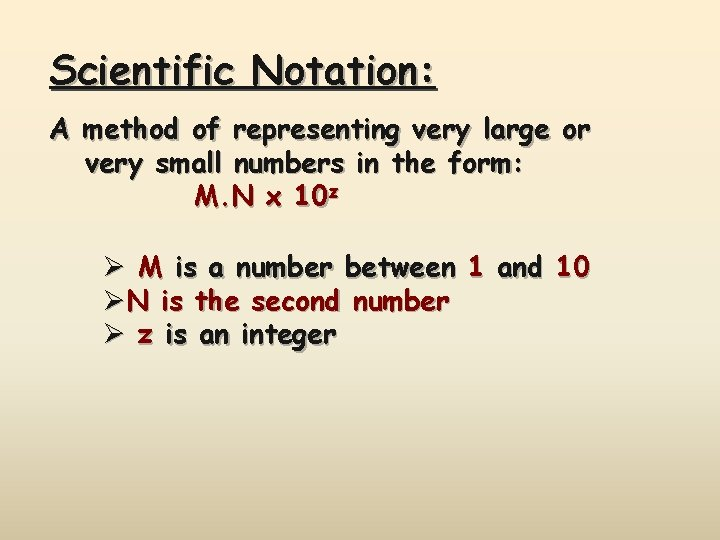Scientific Notation: A method of representing very large or very small numbers in the form: M. N x 10 z Ø M is a number between 1 and 10 ØN is the second number Ø z is an integer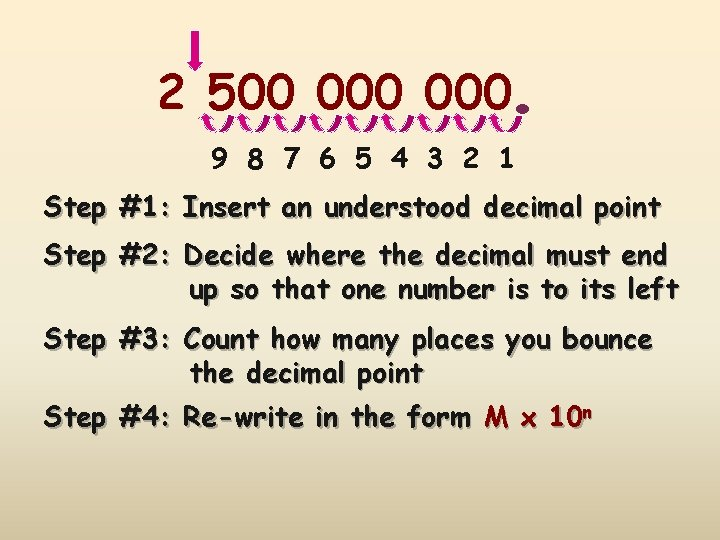. 2 500 000 9 8 7 6 5 4 3 2 1 Step #1: Insert an understood decimal point Step #2: Decide where the decimal must end up so that one number is to its left Step #3: Count how many places you bounce the decimal point Step #4: Re-write in the form M x 10 n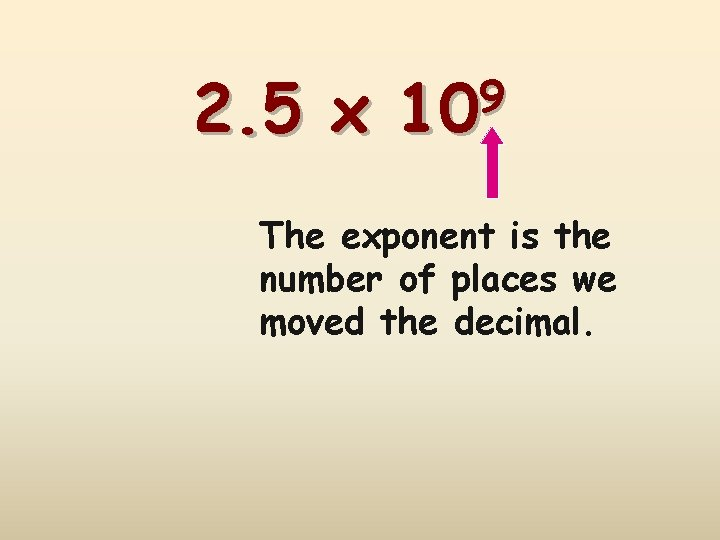2. 5 x 9 10 The exponent is the number of places we moved the decimal.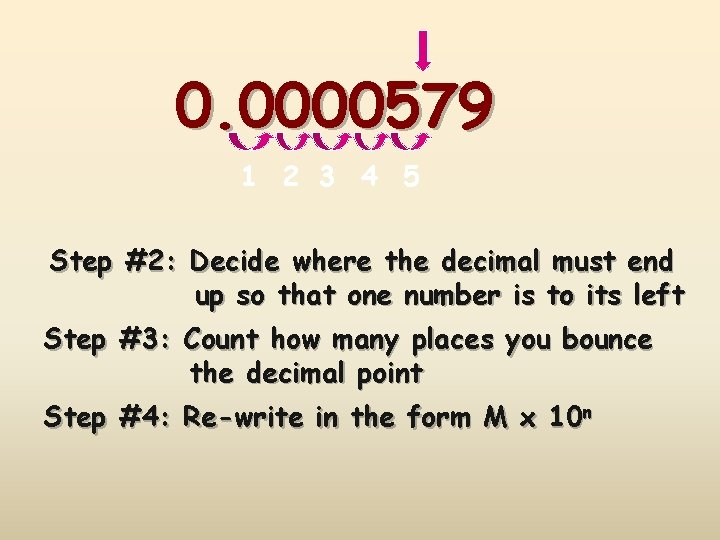0. 0000579 1 2 3 4 5 Step #2: Decide where the decimal must end up so that one number is to its left Step #3: Count how many places you bounce the decimal point Step #4: Re-write in the form M x 10 n5. 79 x -5 10 The exponent is negative because the number we started with was less than 1.PERFORMING CALCULATIONS IN SCIENTIFIC NOTATION ADDITION AND SUBTRACTIONReview: Scientific notation expresses a number in the form: M x 1 M 10 n is an integer4 + 3 7 x x x 106 106 IF the exponents are the same, we simply add or subtract the numbers in front and bring the exponent down unchanged.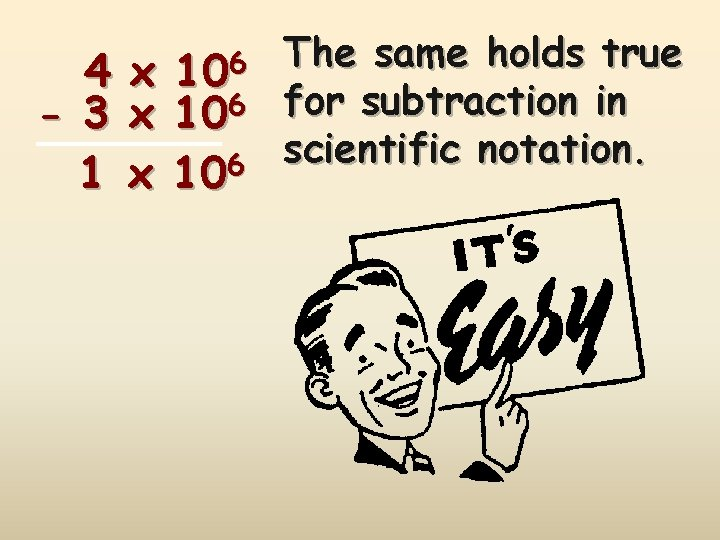4 - 3 1 106 x x x 106 The same holds true for subtraction in scientific notation.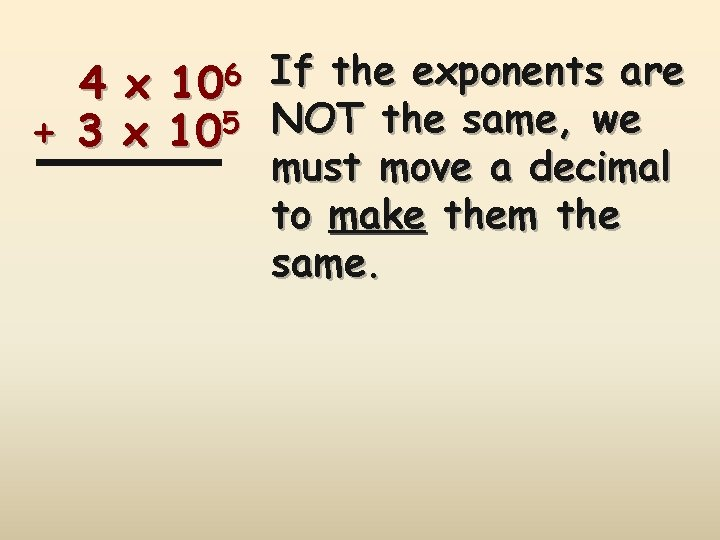4 + 3 106 x x 105 If the exponents are NOT the same, we must move a decimal to make them the same.6 10 4. 00 x 6 5 +. 30 x 10 + 3. 00 x 10 6 4. 30 x 10 Move the decimal on the smaller number! 6 10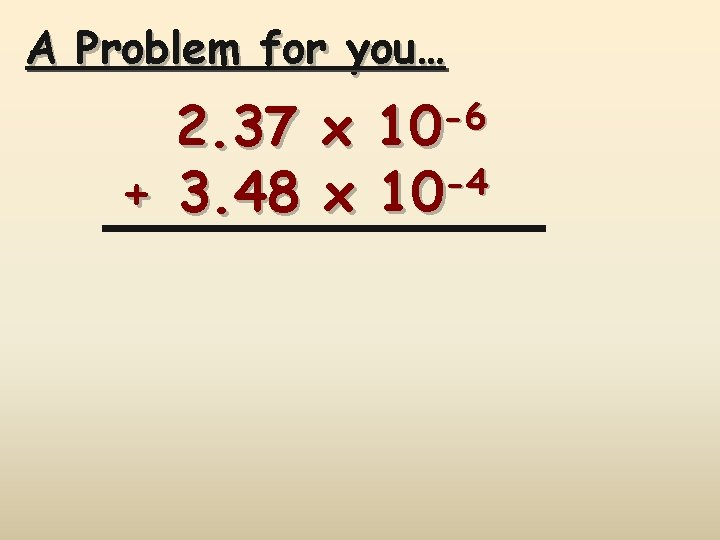A Problem for you… -6 10 2. 37 x -4 + 3. 48 x 10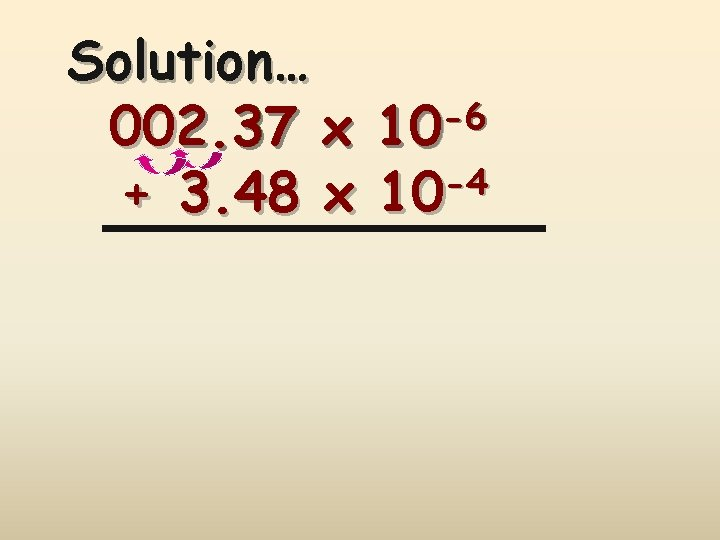Solution… -6 002. 37 x 10 -4 + 3. 48 x 10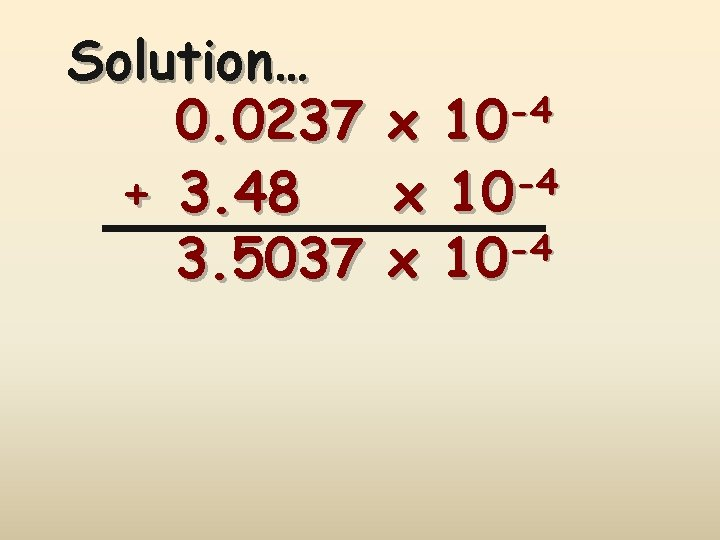Solution… 0. 0237 + 3. 48 3. 5037 -4 10 x -4 x 10